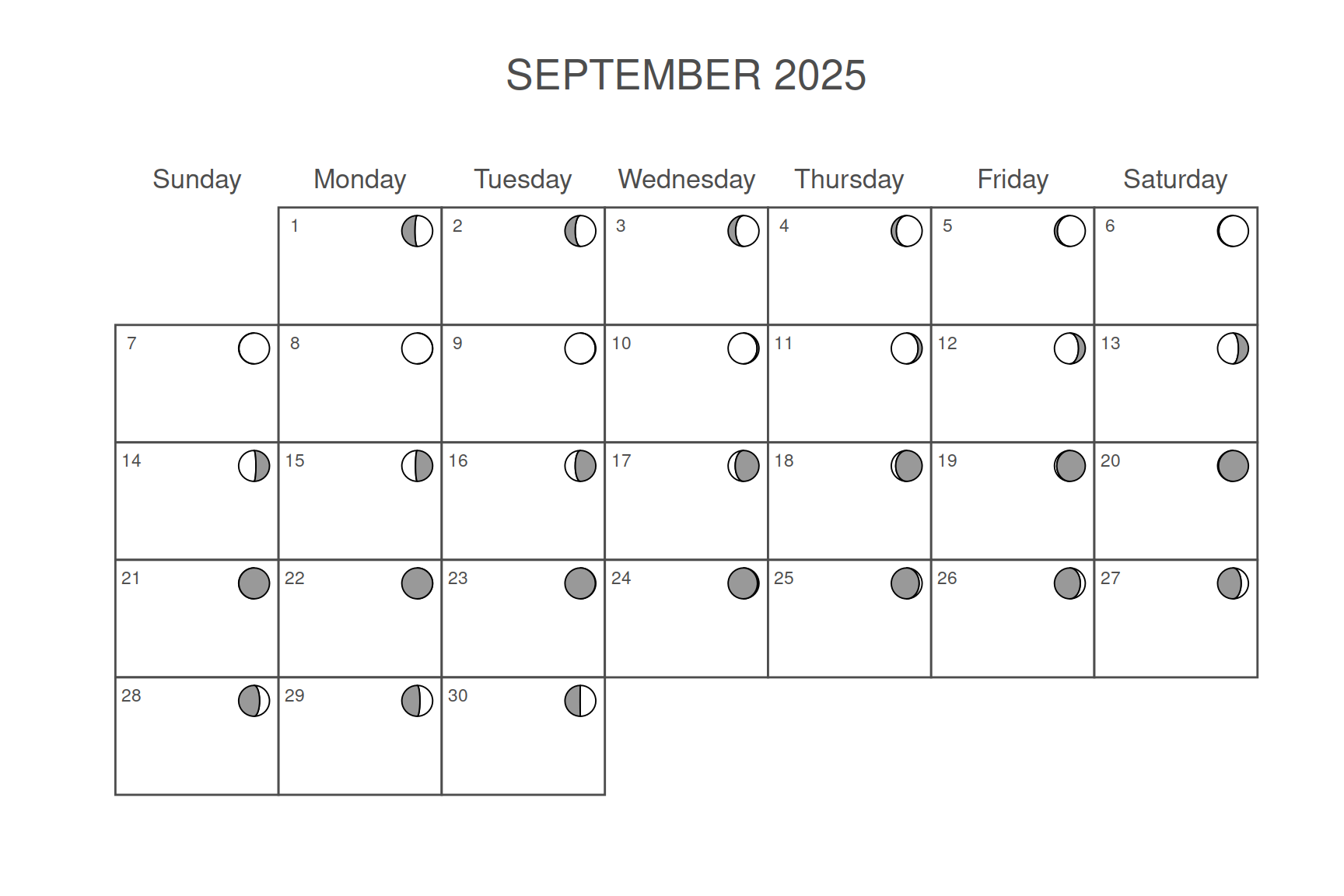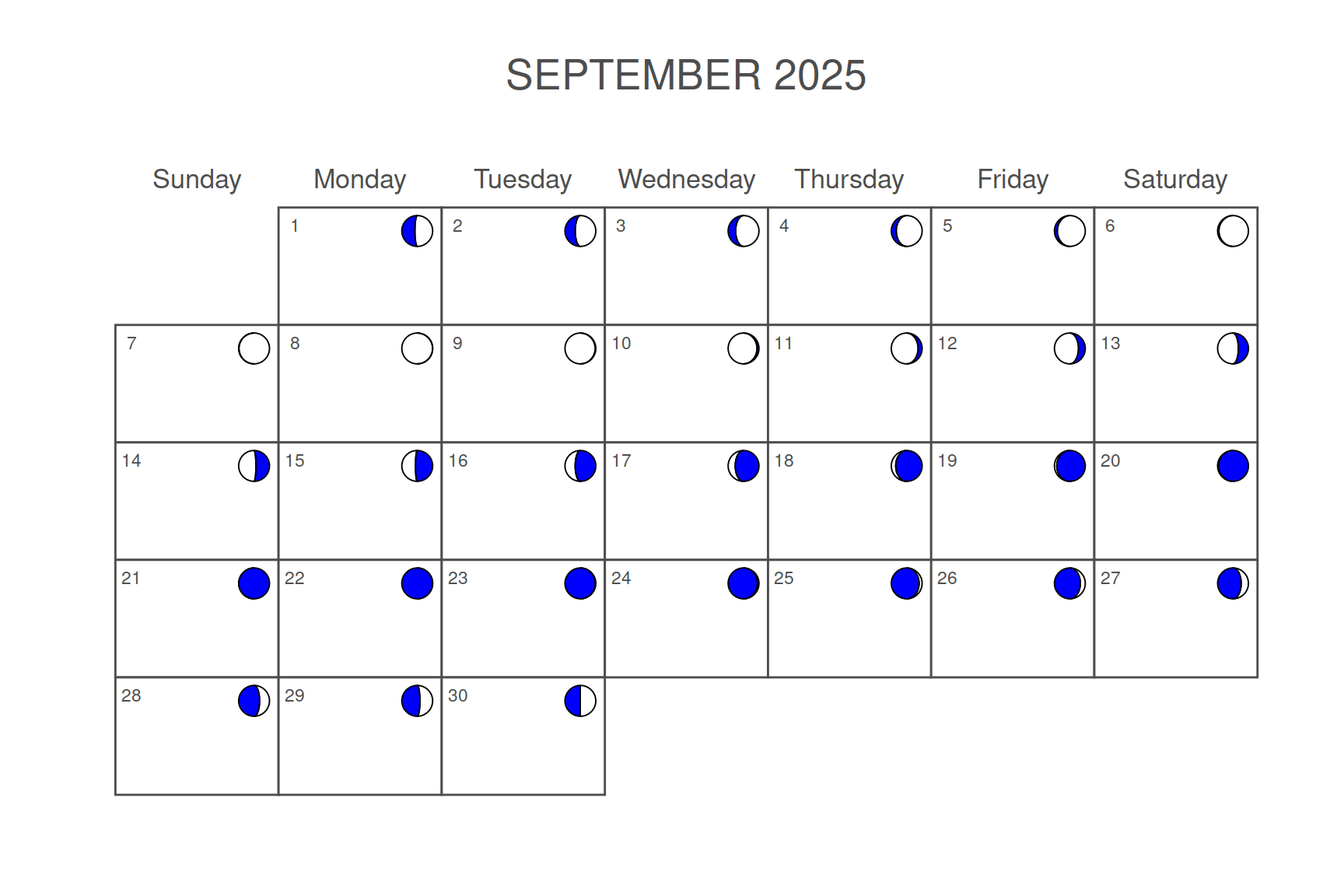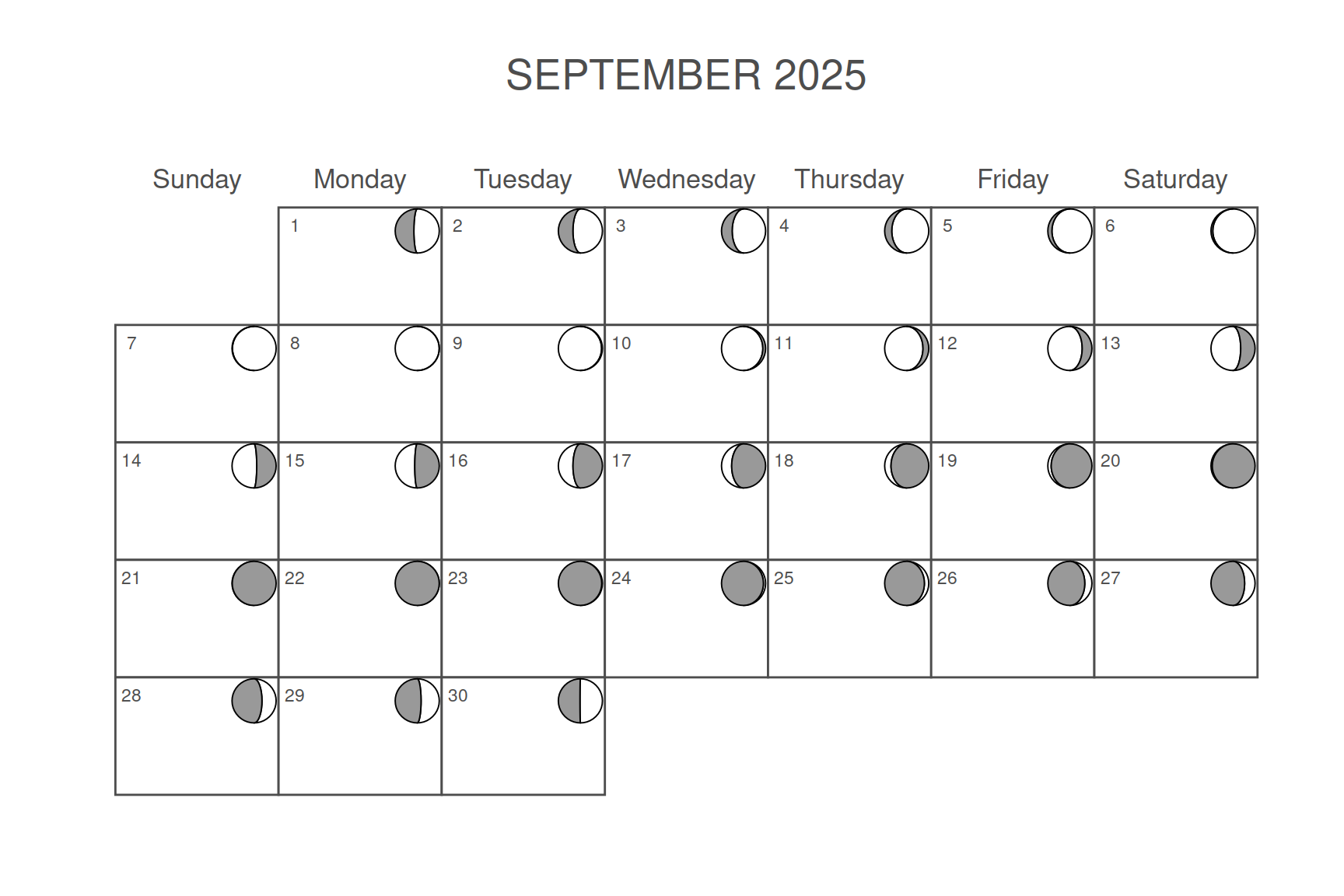# Lunar calendar with ggplot2

R CODER

## Lunar calendar

The `calendR` package allows creating lunar calendars when a month is specified and you set `lunar = TRUE`.

``````# install.packages("calendR")
library(calendR)

calendR(year = 2025,
month = 9,
lunar = TRUE)``````## Color of the moonsThe color of the not visible part of the moons is gray by default, but you can override it with `lunar.col` argument.

``````# install.packages("calendR")
library(calendR)

calendR(year = 2025,
month = 9,
lunar = TRUE,
lunar.col = "blue")``````

## Size of the moons

The size of the moons can also be modified. For that purpose use `lunar.size`. Default value is 7.

``````# install.packages("calendR")
library(calendR)

calendR(year= 2025,
month = 9,
lunar = TRUE,
lunar.size = 10)``````